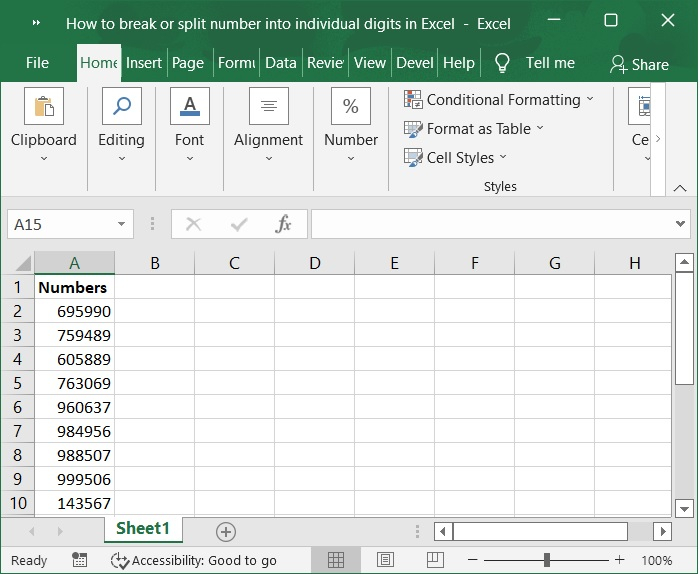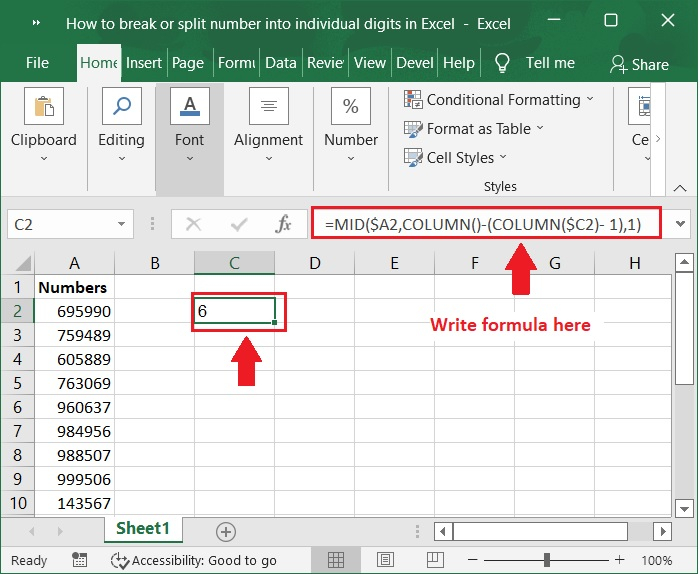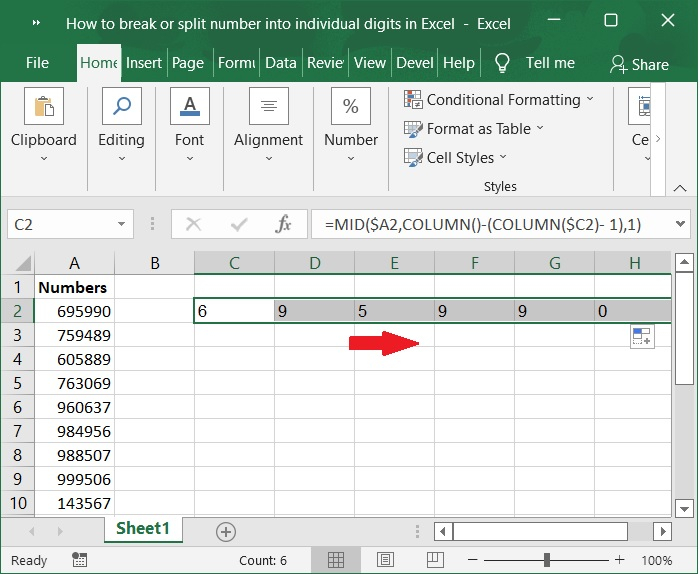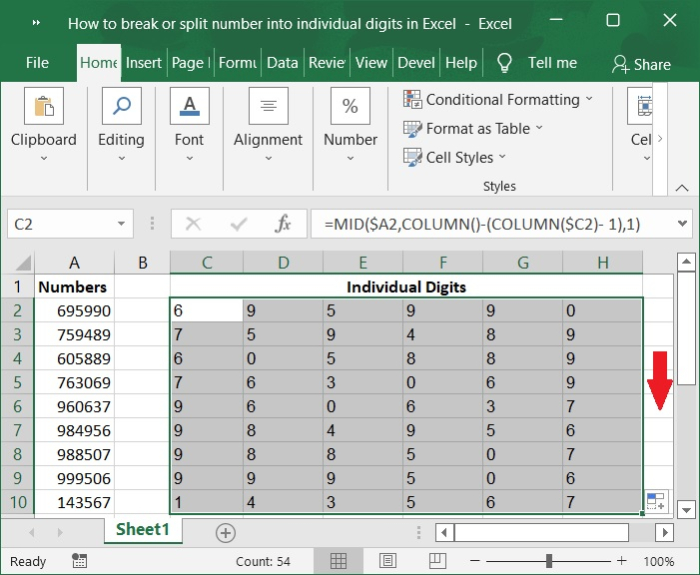# How to break or split number into individual digits in Excel?

Occasionally we might need to separate the numbers from the text or obtain simply the numbers out of any input that contains both text and numbers. Excel has a variety of shortcuts that eliminate the need to manually do this task, making it possible to isolate integers from any dataset. In this tutorial, I will demonstrate a technique for separating nmbers in Excel by using formulas in a variety of circumstances.

## Break or Split Numbers into Individual Digits using Formulas in Excel

In the following part, a formula will be presented that may be used in Excel to split chosen number cells into individual digits.

Let’s understand step by step with an example.

### Step 1

In the first step, let us assume we have a sample data as shown in the below screenshot.### Step 2

To locate the first split digit of the number in cell A2, choose a cell that is blank. In our example cell C2, then type the following formula into the formula bar and then hit the Enter key on your keyboard. Please Refer to the below screenshot for the same.

### Formula

=MID($A2,COLUMN()-(COLUMN($C2)- 1),1)Important Note − In the above formula, the cell with the number that has to be divided into digits is located in A2 and the cell that is used to locate the first split digit is located in C2. Please make the necessary adjustments as per your requirement.

### Step 3

Then, repeat the process of choosing cell C2, then drag the Fill Handle to the right cells until all of the digits in cell A2 have been extracted. Please check out the screenshot below for the same.### Step 4

Maintain the selection of these cells that have split digits, and then drag the Fill Handle down into the cells until all of the numbers are separated into individual digits. Please refer to the below screenshot for same.## Conclusion

In this tutorial, we explained how you can break or split number into individual digits by using formulas in Excel.